## Section 4.6: Graphs of the Other Trigonometric Functions

### Learning Outcomes

• Analyze the graph of  y=tan x and y=cot x.
• Graph variations of  y=tan x and y=cot x.
• Determine a function formula from a tangent or cotangent graph.
• Analyze the graphs of  y=sec x  and  y=csc x.
• Graph variations of  y=sec x  and  y=csc x.
• Determine a function formula from a secant or cosecant graph.

## Analyzing the Graph of y = tan x and Its Variations

We will begin with the graph of the tangent function, plotting points as we did for the sine and cosine functions. Recall that

$\tan x=\frac{\sin x}{\cos x}$

The period of the tangent function is π because the graph repeats itself on intervals of where k is a constant. If we graph the tangent function on $−\dfrac{\pi}{2}\text{ to }\dfrac{\pi}{2}$, we can see the behavior of the graph on one complete cycle. If we look at any larger interval, we will see that the characteristics of the graph repeat.

We can determine whether tangent is an odd or even function by using the definition of tangent.

\begin{align}\tan(−x)&=\frac{\sin(−x)}{\cos(−x)} && \text{Definition of tangent.} \\ &=\frac{−\sin x}{\cos x} && \text{Sine is an odd function, cosine is even.} \\ &=−\frac{\sin x}{\cos x} && \text{The quotient of an odd and an even function is odd.} \\ &=−\tan x && \text{Definition of tangent.} \end{align}

Therefore, tangent is an odd function. We can further analyze the graphical behavior of the tangent function by looking at values for some of the special angles, as listed in the table below.

 x $-\frac{\pi}{6}$ $-\frac{\pi}{3}$ $-\frac{\pi}{4}$ $-\frac{\pi}{6}$ 0 $\frac{\pi}{6}$ $\frac{\pi}{4}$ $\frac{\pi}{3}$ $\frac{\pi}{2}$ tan (x) undefined $−\sqrt{3}$ –1 $−\dfrac{\sqrt{3}}{3}$ 0 $\dfrac{\sqrt{3}}{3}$ 1 $\sqrt{3}$ undefined

These points will help us draw our graph, but we need to determine how the graph behaves where it is undefined. If we look more closely at values when $\frac{\pi}{3}<x<\frac{\pi}{2}$, we can use a table to look for a trend. Because $\frac{\pi}{3}\approx 1.05$ and $\frac{\pi}{2}\approx 1.57$, we will evaluate x at radian measures 1.05 < x < 1.57 as shown in the table below.

 x 1.3 1.5 1.55 1.56 tan x 3.6 14.1 48.1 92.6

As x approaches $\frac{\pi}{2}$, the outputs of the function get larger and larger. Because $y=\tan x$ is an odd function, we see the corresponding table of negative values in the table below.

 x −1.3 −1.5 −1.55 −1.56 tan x −3.6 −14.1 −48.1 −92.6

We can see that, as x approaches $−\dfrac{\pi}{2}$, the outputs get smaller and smaller. Remember that there are some values of x for which cos x = 0. For example, $\cos\left(\frac{\pi}{2}\right)=0$ and $\cos\left(\frac{3\pi}{2}\right)=0$. At these values, the tangent function is undefined, so the graph of $y=\tan x$ has discontinuities at $x=\frac{\pi}{2}$ and $\frac{3\pi}{2}$. At these values, the graph of the tangent has vertical asymptotes. Figure 1 represents the graph of $y=\tan x$. The tangent is positive from 0 to $\frac{\pi}{2}$ and from π to $\frac{3\pi}{2}$, corresponding to quadrants I and III of the unit circle.

## Graphing Variations of y = tan x

As with the sine and cosine functions, the tangent function can be described by a general equation.

$y=A\tan(Bx)$

We can identify horizontal and vertical stretches and compressions using values of A and B. The horizontal stretch can typically be determined from the period of the graph. With tangent graphs, it is often necessary to determine a vertical stretch using a point on the graph.

Because there are no maximum or minimum values of a tangent function, the term amplitude cannot be interpreted as it is for the sine and cosine functions. Instead, we will use the phrase stretching/compressing factor when referring to the constant A.

### A General Note: Features of the Graph of y = Atan(Bx)

• The stretching factor is |A| .
• The period is $P=\frac{\pi}{|B|}$.
• The domain is all real numbers x, where $x\ne \frac{\pi}{2|B|} + \frac{\pi}{|B|} k$ such that k is an integer.
• The range is $\left(-\infty,\infty\right)$.
• The asymptotes occur at $x=\frac{\pi}{2|B|} + \frac{\pi}{|B|}k$, where k is an integer.
• $y = A \tan (Bx)$ is an odd function.

## Graphing One Period of a Stretched or Compressed Tangent Function

We can use what we know about the properties of the tangent function to quickly sketch a graph of any stretched and/or compressed tangent function of the form $f(x)=A\tan(Bx)$. We focus on a single period of the function including the origin, because the periodic property enables us to extend the graph to the rest of the function’s domain if we wish. Our limited domain is then the interval $(−\frac{P}{2}, \frac{P}{2})$ and the graph has vertical asymptotes at $\pm \frac{P}{2}$ where $P=\frac{\pi}{B}$. On $(−\dfrac{\pi}{2}, \dfrac{\pi}{2})$, the graph will come up from the left asymptote at $x=−\dfrac{\pi}{2}$, cross through the origin, and continue to increase as it approaches the right asymptote at $x=\frac{\pi}{2}$. To make the function approach the asymptotes at the correct rate, we also need to set the vertical scale by actually evaluating the function for at least one point that the graph will pass through. For example, we can use

$f\left(\frac{P}{4}\right)=A \tan\left(B\frac{P}{4}\right)=A\tan\left(B\frac{\pi}{4B}\right)=A$

because  $\tan\left(\frac{\pi}{4}\right)=1$.

### How To: Given the function $f(x)=A\tan(Bx)$, graph one period.

1. Identify the stretching factor, |A|.
2. Identify B and determine the period, $P=\frac{\pi}{|B|}$.
3. Draw vertical asymptotes at  $x=−\dfrac{P}{2}$ and $x=\frac{P}{2}$.
4. For A > 0 , the graph approaches the left asymptote at negative output values and the right asymptote at positive output values (reverse for A < 0 ).
5. Plot reference points at $\left(\frac{P}{4},A\right)$ (0, 0), and ($−\dfrac{P}{4}$,− A), and draw the graph through these points.

### Example 1: Sketching a Compressed Tangent

Sketch a graph of one period of the function $y=0.5\tan\left(\frac{\pi}{2}x\right)$.

### Try It

Sketch a graph of $f(x)=3\tan\left(\frac{\pi}{6}x\right)$.

## Graphing One Period of a Shifted Tangent Function

Now that we can graph a tangent function that is stretched or compressed, we will add a vertical and/or horizontal (or phase) shift. In this case, we add C and D to the general form of the tangent function.

$f(x)=A\tan(Bx−C)+D$

The graph of a transformed tangent function is different from the basic tangent function tan x in several ways:

### A General Note: Features of the Graph of $y = A\tan\left(Bx−C\right)+D$

• The stretching factor is |A|.
• The period is $\frac{\pi}{|B|}$.
• The domain is $x\ne\frac{C}{B}+\frac{\pi}{|B|}k$, where k is an integer.
• The range is (−∞,−|A|] ∪ [|A|, ∞).
• The vertical asymptotes occur at $x=\frac{C}{B}+\frac{\pi}{2|B|}k$, where k is an odd integer.
• There is no amplitude.
• $y=A\tan(Bx)$ is an odd function because it is the quotient of odd and even functions (sine and cosine respectively).

### How To: Given the function $y=A\tan(Bx−C)+D$, sketch the graph of one period.

1. Express the function given in the form $y=A\tan(Bx−C)+D$.
2. Identify the stretching/compressing factor, |A|.
3. Identify B and determine the period, $P=\frac{\pi}{|B|}$.
4. Identify C and determine the phase shift, $\frac{C}{B}$.
5. Draw the graph of $y=A\tan(Bx)$ shifted to the right by $\frac{C}{B}$ and up by D.
6. Sketch the vertical asymptotes, which occur at $x=\frac{C}{B}+\frac{\pi}{2|B|}k$, where k is an odd integer.
7. Plot any three reference points and draw the graph through these points.

### Example 2: Graphing One Period of a Shifted Tangent Function

Graph one period of the function $y=−2\tan(\pi x+\pi)−1$.

### Try It

How would the graph in Example 2 look different if we made A = 2 instead of −2?

### How To: Given the graph of a tangent function, identify horizontal and vertical stretches.

1. Find the period P from the spacing between successive vertical asymptotes or x-intercepts.
2. Write $f(x)=A\tan\left(\frac{\pi}{P}x\right)$.
3. Determine a convenient point (x, f(x)) on the given graph and use it to determine A.

### Example 3: Identifying the Graph of a Stretched Tangent

Find a formula for the function graphed in Figure 5.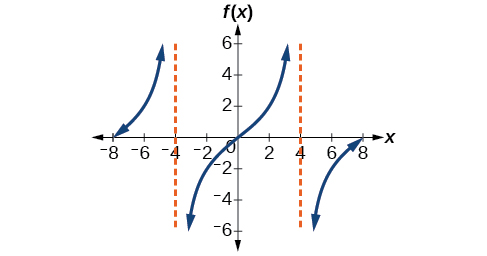Figure 5

### Try It

Find a formula for the function in Figure 6.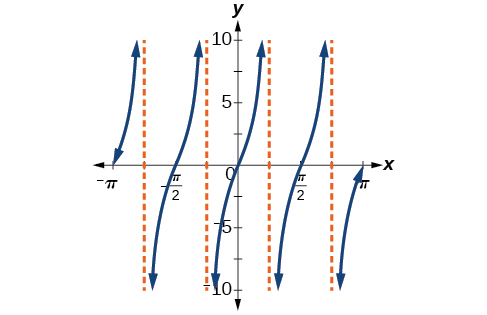Figure 6

## Using the Graphs of Trigonometric Functions to Solve Real-World Problems

Many real-world scenarios represent periodic functions and may be modeled by trigonometric functions. As an example, let’s return to the scenario from the section opener. Have you ever observed the beam formed by the rotating light on a police car and wondered about the movement of the light beam itself across the wall? The periodic behavior of the distance the light shines as a function of time is obvious, but how do we determine the distance? We can use the tangent function .

### Example 4: Using Trigonometric Functions to Solve Real-World Scenarios

Suppose the function $y=5\tan\left(\frac{\pi}{4}t\right)$ marks the distance in the movement of a light beam from the top of a police car across a wall where t is the time in seconds and y is the distance in feet from a point on the wall directly across from the police car.

1. Find and interpret the stretching factor and period.
2. Graph on the interval [0, 5].
3. Evaluate f(1) and discuss the function’s value at that input.

## Analyzing the Graphs of y = sec x and y = cscx and Their Variations

The secant was defined by the reciprocal identity $\sec x=\frac{1}{\cos x}$. Notice that the function is undefined when the cosine is 0, leading to vertical asymptotes at $\frac{\pi}{2},\frac{3\pi}{2}\text{, etc}$. Because the cosine is never more than 1 in absolute value, the secant, being the reciprocal, will never be less than 1 in absolute value.

We can graph $y=\sec x$ by observing the graph of the cosine function because these two functions are reciprocals of one another. See Figure 9. The graph of the cosine is shown as a dashed orange wave so we can see the relationship. Where the graph of the cosine function decreases, the graph of the secant function increases. Where the graph of the cosine function increases, the graph of the secant function decreases. When the cosine function is zero, the secant is undefined.

The secant graph has vertical asymptotes at each value of x where the cosine graph crosses the x-axis; we show these in the graph below with dashed vertical lines, but will not show all the asymptotes explicitly on all later graphs involving the secant and cosecant.

Note that, because cosine is an even function, secant is also an even function. That is, $\sec(−x)=\sec x$.

As we did for the tangent function, we will again refer to the constant |A| as the stretching factor, not the amplitude.

### A General Note: Features of the Graph of y = Asec(Bx)

• The stretching factor is |A|.
• The period is $\frac{2\pi}{|B|}$.
• The domain is $x\ne \frac{\pi}{2|B|}k$, where k is an odd integer.
• The range is (−∞, −|A|] ∪ [|A|, ∞).
• The vertical asymptotes occur at $x=\frac{\pi}{2|B|}k$, where k is an odd integer.
• There is no amplitude.
• $y=A\sec(Bx)$ is an even function because cosine is an even function.

Similar to the secant, the cosecant is defined by the reciprocal identity $\csc x=1\sin x$. Notice that the function is undefined when the sine is 0, leading to a vertical asymptote in the graph at 0, π, etc. Since the sine is never more than 1 in absolute value, the cosecant, being the reciprocal, will never be less than 1 in absolute value.

We can graph $y=\csc x$ by observing the graph of the sine function because these two functions are reciprocals of one another. See Figure 10. The graph of sine is shown as a dashed orange wave so we can see the relationship. Where the graph of the sine function decreases, the graph of the cosecant function increases. Where the graph of the sine function increases, the graph of the cosecant function decreases.

The cosecant graph has vertical asymptotes at each value of x where the sine graph crosses the x-axis; we show these in the graph below with dashed vertical lines.

Note that, since sine is an odd function, the cosecant function is also an odd function. That is, $\csc(−x)=−\csc x$.

The graph of cosecant, which is shown in Figure 10, is similar to the graph of secant.

### A General Note: Features of the Graph of $y=A\csc(Bx) • The stretching factor is |A|. • The period is [latex]\frac{2\pi}{|B|}$.
• The domain is $x\ne\frac{\pi}{|B|}k$, where k is an integer.
• The range is ( −∞, −|A|] ∪ [|A|, ∞).
• The asymptotes occur at $x=\frac{\pi}{|B|}k$, where k is an integer.
• $y=A\csc(Bx)$ is an odd function because sine is an odd function.

## Graphing Variations of y = sec x and y = csc x

For shifted, compressed, and/or stretched versions of the secant and cosecant functions, we can follow similar methods to those we used for tangent and cotangent. That is, we locate the vertical asymptotes and also evaluate the functions for a few points (specifically the local extrema). If we want to graph only a single period, we can choose the interval for the period in more than one way. The procedure for secant is very similar, because the cofunction identity means that the secant graph is the same as the cosecant graph shifted half a period to the left. Vertical and phase shifts may be applied to the cosecant function in the same way as for the secant and other functions. The equations become the following.

$y=A\sec(Bx−C)+D$
$y=A\csc(Bx−C)+D$

### A General Note: Features of the Graph of $y=A\sec(Bx−C)+D$

• The stretching factor is |A|.
• The period is $\frac{2\pi}{|B|}$.
• The domain is $x\ne \frac{C}{B}+\frac{\pi}{2|B|}k$, where k is an odd integer.
• The range is (−∞, −|A|] ∪ [|A|, ∞).
• The vertical asymptotes occur at $x=\frac{C}{B}+\frac{\pi}{2|B|}k$, where k is an odd integer.
• There is no amplitude.
• $y=A\sec(Bx)$ is an even function because cosine is an even function.

### A General Note: Features of the Graph of $y=A\csc(Bx−C)+D$

• The stretching factor is |A|.
• The period is $\frac{2\pi}{|B|}$.
• The domain is $x\ne\frac{C}{B}+\frac{\pi}{2|B|}k$, where k is an integer.
• The range is (−∞, −|A|] ∪ [|A|, ∞).
• The vertical asymptotes occur at $x=\frac{C}{B}+\frac{\pi}{|B|}k$, where k is an integer.
• There is no amplitude.
• $y=A\csc(Bx)$ is an odd function because sine is an odd function.

### How To: Given a function of the form $y=A\sec(Bx)$, graph one period.

1. Express the function given in the form $y=A\sec(Bx)$.
2. Identify the stretching/compressing factor, |A|.
3. Identify B and determine the period, $P=\frac{2\pi}{|B|}$.
4. Sketch the graph of $y=A\cos(Bx)$.
5. Use the reciprocal relationship between $y=\cos x$ and $y=\sec x$ to draw the graph of $y=A\sec(Bx)$.
6. Sketch the asymptotes.
7. Plot any two reference points and draw the graph through these points.

### Example 6: Graphing a Variation of the Secant Function

Graph one period of $f(x)=2.5\sec(0.4x)$.

### Try It

Graph one period of $f(x)=−2.5\sec(0.4x)$.

### Do the vertical shift and stretch/compression affect the secant’s range?

Yes. The range of $f(x) = A\sec(Bx − C) + D$ is ( −∞, −|A| + D] ∪ [|A| + D, ∞).

### How To: Given a function of the form $f(x)=A\sec (Bx−C)+D$, graph one period.

1. Express the function given in the form $y=A\sec(Bx−C)+D$.
2. Identify the stretching/compressing factor, |A|.
3. Identify B and determine the period, $\frac{2\pi}{|B|}$.
4. Identify C and determine the phase shift, $\frac{C}{B}$.
5. Draw the graph of $y=A\sec(Bx)$. but shift it to the right by $\frac{C}{B}$ and up by D.
6. Sketch the vertical asymptotes, which occur at $x=\frac{C}{B}+\frac{\pi}{2|B|}k$, where k is an odd integer.

### Example 7: Graphing a Variation of the Secant Function

Graph one period of $y=4\sec \left(\frac{\pi}{3}x−\frac{\pi}{2}\right)+1$.

### Try It

Graph one period of $f(x)=−6\sec(4x+2)−8$.

### Q & A

#### The domain of $\csc x$ was given to be all x such that $x\ne k\pi$ for any integer k. Would the domain of $y=A\csc(Bx−C)+D$ be $x\ne\frac{C+k\pi}{B}$?

Yes. The excluded points of the domain follow the vertical asymptotes. Their locations show the horizontal shift and compression or expansion implied by the transformation to the original function’s input.

### How To: Given a function of the form $y=A\csc(Bx)$, graph one period.

1. Express the function given in the form $y=A\csc(Bx)$.
2. |A|.
3. Identify B and determine the period, $P=\frac{2\pi}{|B|}$.
4. Draw the graph of $y=A\sin(Bx)$.
5. Use the reciprocal relationship between $y=\sin x$ and $y=\csc x$ to draw the graph of $y=A\csc(Bx)$.
6. Sketch the asymptotes.
7. Plot any two reference points and draw the graph through these points.

### Example 8: Graphing a Variation of the Cosecant Function

Graph one period of $f(x)=−3\csc(4x)$.

### Try It

Graph one period of $f(x)=0.5\csc(2x)$.

### How To: Given a function of the form $f(x)=A\csc(Bx−C)+D$, graph one period.

1. Express the function given in the form $y=A\csc(Bx−C)+D$.
2. Identify the stretching/compressing factor, |A|.
3. Identify B and determine the period, $\frac{2\pi}{|B|}$.
4. Identify C and determine the phase shift, $\frac{C}{B}$.
5. Draw the graph of $y=A\csc(Bx)$ but shift it to the right by and up by D.
6. Sketch the vertical asymptotes, which occur at $x=\frac{C}{B}+\frac{\pi}{|B|}k$, where k is an integer.

### Example 9: Graphing a Vertically Stretched, Horizontally Compressed, and Vertically Shifted Cosecant

Sketch a graph of $y=2\csc\left(\frac{\pi}{2}x\right)+1$. What are the domain and range of this function?

### Try It

Given the graph of $f(x)=2\cos\left(\frac{\pi}{2}x\right)+1$ shown in Figure 15, sketch the graph of $g(x)=2\sec\left(\frac{\pi}{2}x\right)+1$ on the same axes.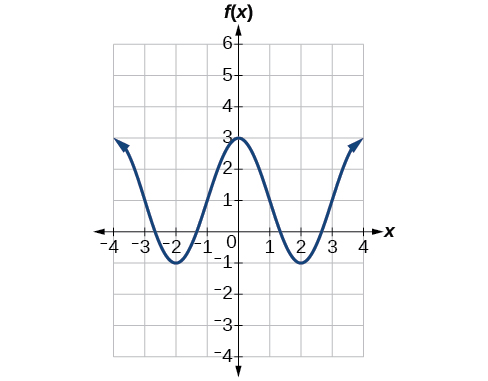Figure 15

## Analyzing the Graph of y = cot x and Its Variations

The last trigonometric function we need to explore is cotangent. The cotangent is defined by the reciprocal identity $\cot x=\frac{1}{\tan x}$. Notice that the function is undefined when the tangent function is 0, leading to a vertical asymptote in the graph at 0, π, etc. Since the output of the tangent function is all real numbers, the output of the cotangent function is also all real numbers.

We can graph $y=\cot x$ by observing the graph of the tangent function because these two functions are reciprocals of one another. See Figure 16. Where the graph of the tangent function decreases, the graph of the cotangent function increases. Where the graph of the tangent function increases, the graph of the cotangent function decreases.

The cotangent graph has vertical asymptotes at each value of x where $\tan x=0$; we show these in the graph below with dashed lines. Since the cotangent is the reciprocal of the tangent, $\cot x$ has vertical asymptotes at all values of x where $\tan x=0$ , and $\cot x=0$ at all values of x where tan x has its vertical asymptotes.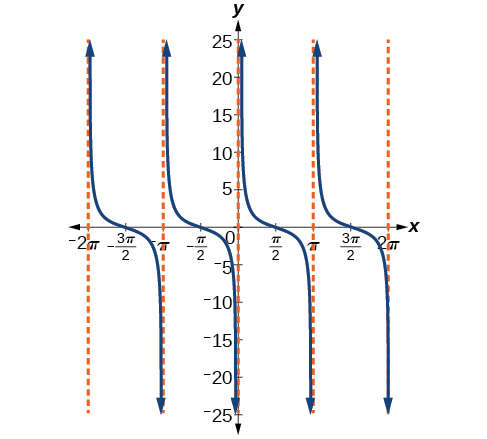### A General Note: Features of the Graph of y = Acot(Bx)

• The stretching factor is |A|.
• The period is $P=\frac{\pi}{|B|}$.
• The domain is $x\ne\frac{\pi}{|B|}k$, where k is an integer.
• The range is (−∞, ∞).
• The asymptotes occur at $x=\frac{\pi}{|B|}k$, where k is an integer.
• $y=A\cot(Bx)$ is an odd function.

## Graphing Variations of y = cot x

We can transform the graph of the cotangent in much the same way as we did for the tangent. The equation becomes the following.

$y=A\cot(Bx−C)+D$

### A General Note: Properties of the Graph of y = Acot(Bx−C)+D

• The stretching factor is |A|.
• The period is $\frac{\pi}{|B|}$.
• The domain is $x\ne\frac{C}{B}+\frac{\pi}{|B|}k$, where k is an integer.
• The range is (−∞, −|A|] ∪ [|A|, ∞).
• The vertical asymptotes occur at $x=\frac{C}{B}+\frac{\pi}{|B|}k$, where k is an integer.
• There is no amplitude.
• $y=A\cot(Bx)$ is an odd function because it is the quotient of even and odd functions (cosine and sine, respectively)

### How To: Given a modified cotangent function of the form $f(x)=A\cot(Bx)$, graph one period.

1. Express the function in the form $f(x)=A\cot(Bx)$.
2. Identify the stretching factor, |A|.
3. Identify the period, $P=\frac{\pi}{|B|}$.
4. Draw the graph of $y=A\tan(Bx)$.
5. Plot any two reference points.
6. Use the reciprocal relationship between tangent and cotangent to draw the graph of $y=A\cot(Bx)$.
7. Sketch the asymptotes.

### Example 10: Graphing Variations of the Cotangent Function

Determine the stretching factor, period, and phase shift of $y=3\cot(4x)$, and then sketch a graph.

### How To: Given a modified cotangent function of the form $f(x)=A\cot(Bx−C)+D$, graph one period.

1. Express the function in the form $f(x)=A\cot(Bx−C)+D$.
2. Identify the stretching factor, |A|.
3. Identify the period, $P=\frac{\pi}{|B|}$.
4. Identify the phase shift, $\frac{C}{B}$.
5. Draw the graph of $y=A\tan(Bx)$ shifted to the right by $\frac{C}{B}$ and up by D.
6. Sketch the asymptotes $x =\frac{C}{B}+\frac{\pi}{|B|}k$, where k is an integer.
7. Plot any three reference points and draw the graph through these points.

### Example 11: Graphing a Modified Cotangent

Sketch a graph of one period of the function $f(x)=4\cot\left(\frac{\pi}{8}x−\frac{\pi}{2}\right)−2$.

## Key Equations

 Shifted, compressed, and/or stretched tangent function $y=A\tan(Bx−C)+D$ Shifted, compressed, and/or stretched secant function $y=A\sec(Bx−C)+D$ Shifted, compressed, and/or stretched cosecant $y=A\csc(Bx−C)+D$ Shifted, compressed, and/or stretched cotangent function $y=A\cot(Bx−C)+D$

## Key Concepts

• The tangent function has period π.
• $f(x)=A\tan(Bx−C)+D$ is a tangent with vertical and/or horizontal stretch/compression and shift.
• The secant and cosecant are both periodic functions with a period of2π. $f(x)=A\sec(Bx−C)+D$ gives a shifted, compressed, and/or stretched secant function graph.
• $f(x)=A\csc(Bx−C)+D$ gives a shifted, compressed, and/or stretched cosecant function graph.
• The cotangent function has period π and vertical asymptotes at 0, ±π,±2π,….
• The range of cotangent is (−∞,∞),and the function is decreasing at each point in its range.
• The cotangent is zero at $\pm\frac{\pi}{2}\text{, }\pm\frac{3\pi}{2}$,….
• $f(x)=A\cot(Bx−C)+D$ is a cotangent with vertical and/or horizontal stretch/compression and shift.
• Real-world scenarios can be solved using graphs of trigonometric functions.

## Section 4.6 Homework Exercises

1. Explain how the graph of the sine function can be used to graph $y=\csc x$.

2. How can the graph of $y=\cos x$ be used to construct the graph of $y=\sec x$?

3. Explain why the period of $\tan x$ is equal to π.

4. Why are there no intercepts on the graph of $y=\csc x$?

5. How does the period of $y=\csc x$ compare with the period of $y=\sin x$?

For the following exercises, match each trigonometric function with one of the following graphs.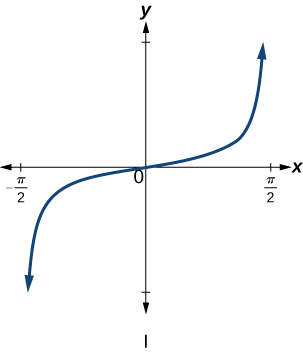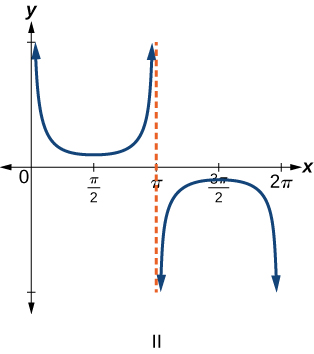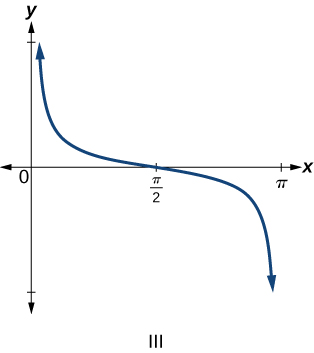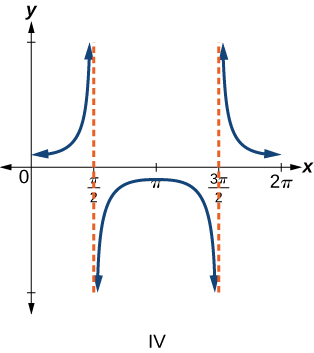6. $f(x)=\tan x$

7. $f(x)=\sec x$

8. $f(x)=\csc x$

9. $f(x)=\cot x$

For the following exercises, find the period and horizontal shift of each of the functions.

10. $f(x)=2\tan(4x−32)$

11. $h(x)=2\sec\left(\frac{\pi}{4}(x+1)\right)$

12. $m(x)=6\csc\left(\frac{\pi}{3}x+\pi\right)$

13. If tan x = −1.5, find tan(−x).

14. If sec x = 2, find sec(−x).

15. If csc x = −5, find csc(−x).

16. If $x\sin x=2$, find $(−x)\sin(−x)$.

For the following exercises, rewrite each expression such that the argument x is positive.

17. $\cot(−x)\cos(−x)+\sin(−x)$

18. $\cos(−x)+\tan(−x)\sin(−x)$

For the following exercises, sketch two periods of the graph for each of the following functions. Identify the stretching factor, period, and asymptotes.

19. $f(x)=2\tan(4x−32)$

20. $h(x)=2\sec\left(\frac{\pi}{4}\left(x+1\right)\right)$

21. $m(x)=6\csc\left(\frac{\pi}{3}x+\pi\right)$

22. $j(x)=\tan\left(\frac{\pi}{2}x\right)$

23. $p(x)=\tan\left(x−\frac{\pi}{2}\right)$

24. $f(x)=4\tan(x)$

25. $f(x)=\tan\left(x+\frac{\pi}{4}\right)$

26. $f(x)=\pi\tan\left(\pi x−\pi\right)−\pi$

27. $f(x)=2\csc(x)$

28. $f(x)=−\frac{1}{4}\csc(x)$

29. $f(x)=4\sec(3x)$

30. $f(x)=−3\cot(2x)$

31. $f(x)=7\sec(5x)$

32. $f(x)=\frac{9}{10}\csc(\pi x)$

33. $f(x)=2\csc \left(x+\frac{\pi}{4}\right)−1$

34. $f(x)=−\sec \left(x−\frac{\pi}{3}\right)−2$

35. $f(x)=\frac{7}{5}\csc \left(x−\frac{\pi}{4}\right)$

36. $f(x)=5\left(\cot\left(x+\frac{\pi}{2}\right)−3\right)$

For the following exercises, find and graph two periods of the periodic function with the given stretching factor, |A|, period, and phase shift.

37. A tangent curve, $A=1$, period of $\frac{\pi}{3}$; and phase shift $(h\text{,}k)=\left(\frac{\pi}{4}\text{,}2\right)$

38. A tangent curve, $A=−2$, period of $\frac{\pi}{4}$, and phase shift $(h\text{,}k)=\left(−\frac{\pi}{4}\text{,}−2\right)$

For the following exercises, find an equation for the graph of each function.

39.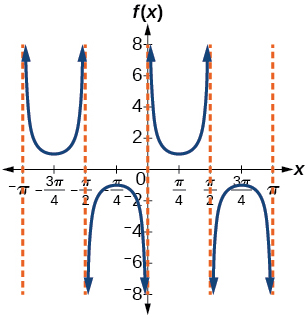40.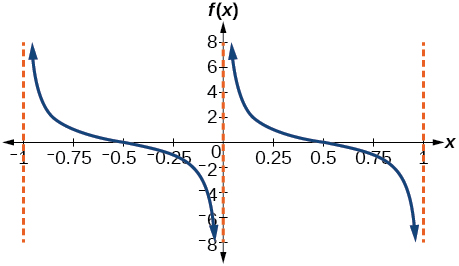41.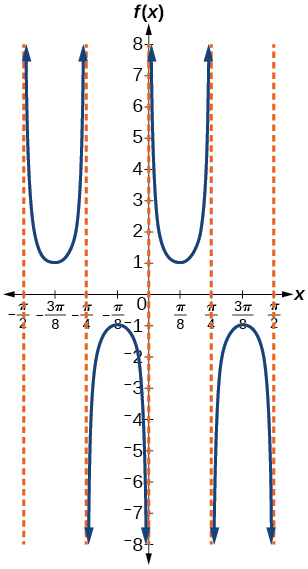42.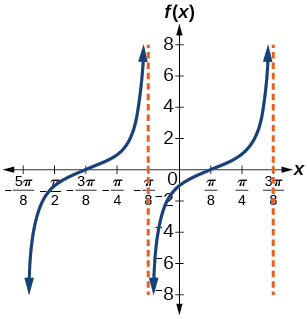43.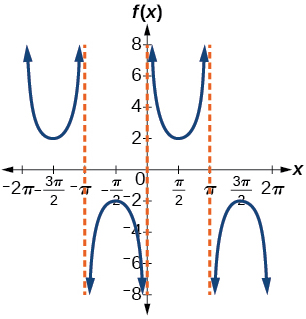44.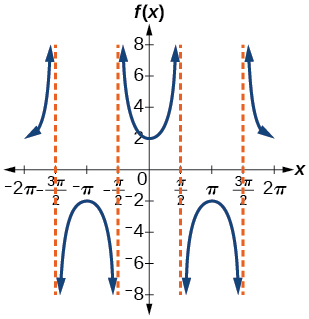45.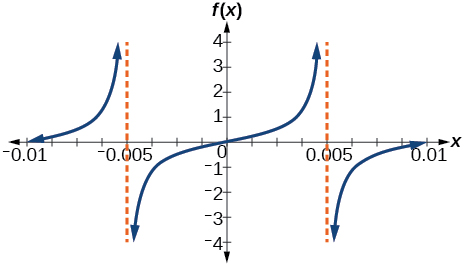47.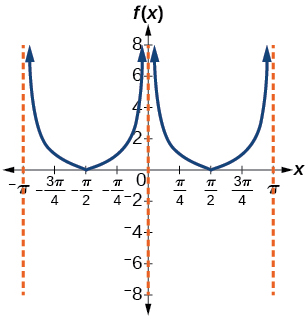49.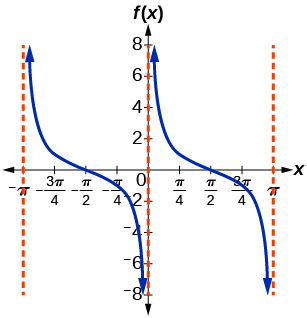50. Graph $f(x)=1+\sec^{2}(x)−\tan^{2}(x)$. What is the function shown in the graph?

51. $f(x)=\sec(0.001x)$

52. $f(x)=\cot(100\pi x)$

53. $f(x)=\sin^{2}x+\cos^{2}x$

54. The function $f(x)=20\tan\left(\frac{\pi}{10}x\right)$ marks the distance in the movement of a light beam from a police car across a wall for time x, in seconds, and distance $f(x)$, in feet.

a. Graph on the interval[0,5].
b. Find and interpret the stretching factor, period, and asymptote.
c. Evaluate f(1) and f(2.5) and discuss the function’s values at those inputs.

55. Standing on the shore of a lake, a fisherman sights a boat far in the distance to his left. Let x, measured in radians, be the angle formed by the line of sight to the ship and a line due north from his position. Assume due north is 0 and x is measured negative to the left and positive to the right. (See Figure 19.) The boat travels from due west to due east and, ignoring the curvature of the Earth, the distance $d(x)$, in kilometers, from the fisherman to the boat is given by the function $d(x)=1.5\sec(x)$.

a. What is a reasonable domain for $d(x)$?
b. Graph d(x) on this domain.
c. Find and discuss the meaning of any vertical asymptotes on the graph of $d(x)$.
d. Calculate and interpret $d(−\frac{\pi}{3})$. Round to the second decimal place.
e. Calculate and interpret $d(\frac{\pi}{6})$. Round to the second decimal place.
f. What is the minimum distance between the fisherman and the boat? When does this occur?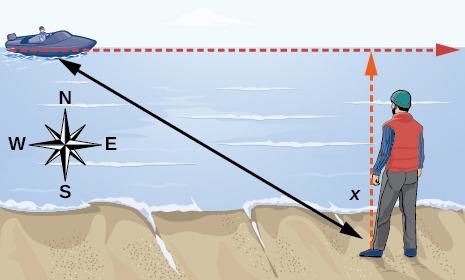Figure 19

56. A laser rangefinder is locked on a comet approaching Earth. The distance $g(x)$, in kilometers, of the comet after x days, for x in the interval 0 to 30 days, is given by $g(x)=250,000\csc\left(\frac{\pi}{30}x\right)$.

a. Graph $g(x)$ on the interval [0,35].
b. Evaluate $g(5)$ and interpret the information.
c. What is the minimum distance between the comet and Earth? When does this occur? To which constant in the equation does this correspond?
d. Find and discuss the meaning of any vertical asymptotes.

57. A video camera is focused on a rocket on a launching pad 2 miles from the camera. The angle of elevation from the ground to the rocket after x seconds is $\frac{\pi}{120}x$.

a. Write a function expressing the altitude $h(x)$, in miles, of the rocket above the ground after x seconds. Ignore the curvature of the Earth.
b. Graph $h(x)$ on the interval (0,60).
c. Evaluate and interpret the values $h(0)$ and $h(30)$.
d. What happens to the values of $h(x)$ as x approaches 60 seconds? Interpret the meaning of this in terms of the problem.